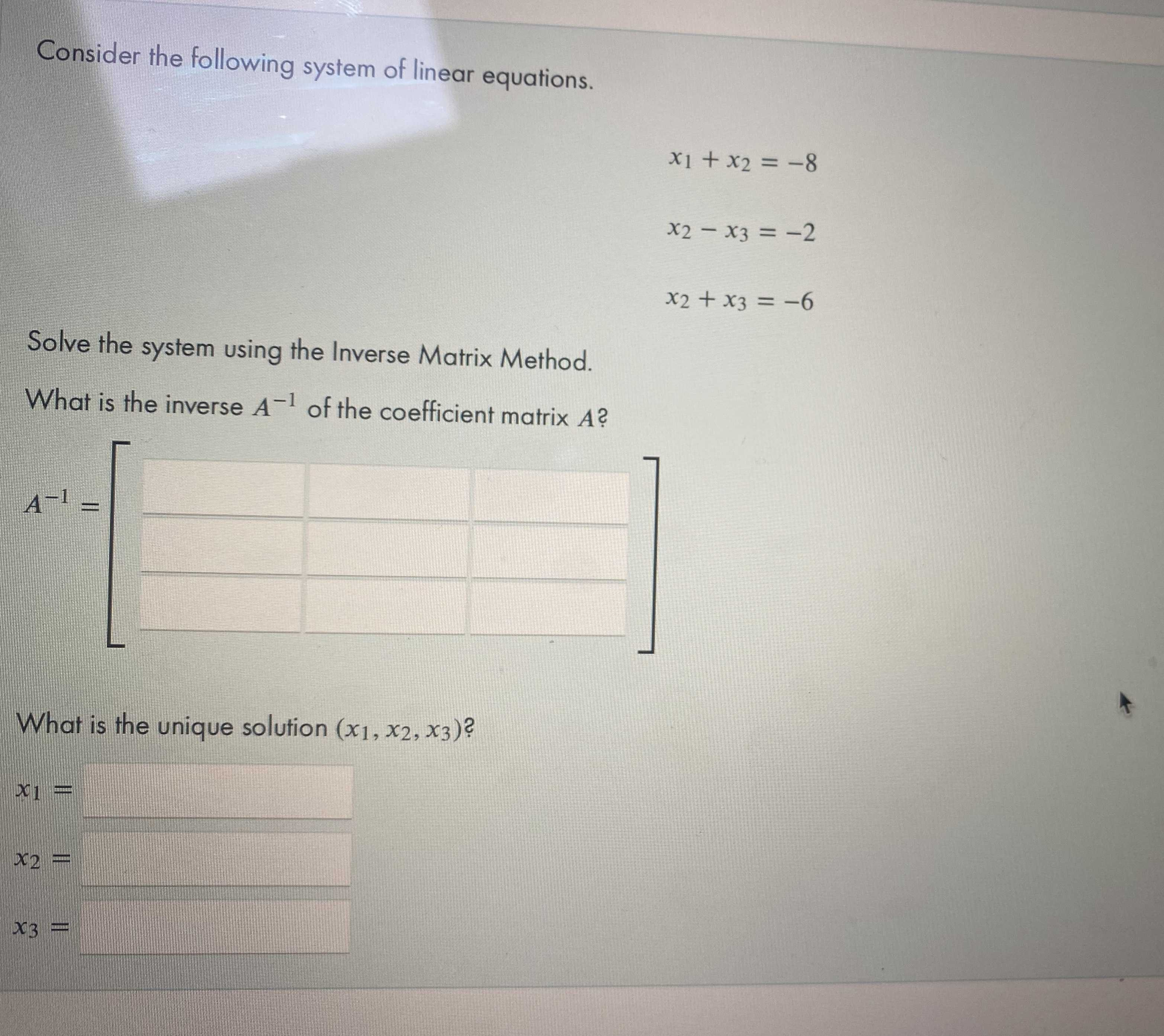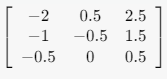### Still have math questions?

Algebra
QuestionConsider the following system of linear equations.

$$x _ { 1 } + x _ { 2 } = - 8$$ $$x _ { 2 } - x _ { 3 } = - 2$$ $$x _ { 2 } + x _ { 3 } = - 6$$

Solve the system using the Inverse Matrix Method. What is the inverse $$A ^ { - 1 }$$ of the coefficient matrix $$A ?$$

$$A ^ { - 1 } = - 2$$

$$x _ { 3 } =$$

$$x _ { 2 } =$$